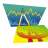# Choppiness Indicator for ThinkorSwim

T

#### tomsk

##### Well-known member
VIP
For those interested, here is a Choppiness Indicator that Mobius posted several years ago.
This would be helpful for those who'd like another measure of defining trend, chop, etc

Code:
``````# Choppiness Indicator
# Mobius
# V001.03.2012

#Hint: The Market is considered TRENDING when the index is below 38.2 \n The Market is considered CHOPPY when the index is above 61.8. \n A move above the 38.2 Level indicates a possible end to a trend and a move below 61.8 indicates a possible breakout from a period of consolidation.

declare lower;
input Length   = 14;
input Signal   =  3;
input Choppy   = 62;
input MidLine  = 50;
input Trending = 38;
input CIx = {default CIB, CIA};
def CIA = 100 * log( Sum( TrueRange(high, close, low), Length))
/ ( Highest(close, Length) - Lowest(close, Length))
/ log(Length);
def CIB = ((log(Sum(TrueRange(high, close, low), Length) /
(Highest(if high >= close then high else
close, Length) -
Lowest( if low <= close then low else close, Length)))
/ log(10)) / (log(Length)/log(10))) * 100;
plot CI = if CIx == CIx.CIB then CIB else CIA;
CI.SetDefaultColor( Color.White);
plot CIavg = average(CI, Signal);;
CIavg.setdefaultColor(Color.Green);
plot Chop = Choppy;
Chop.SetDefaultColor(Color.red);
plot Mid = MidLine;
Mid.SetDefaultColor(Color.gray);
plot Trend = Trending;
Trend.SetDefaultColor(Color.green);
AddLabel(yes, if CI > MidLine then "CHOPPY " else "TRENDING ", if CI < Trend then Color.Green else if CI > Trend and CI < MidLine then Color.Light_Green else if CI > MidLine and CI < Chop then Color.Yellow else Color.Red);Easycator's VWAP Trading Indicator/Strategy Questions 8